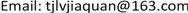——以Z市为例1. 引言

“城镇化”是指农村人口不断向城镇转移，第二、三产业不断向城镇聚集，从而使城镇数量增加、规模扩大的一种历史过程。城镇化问题是我国经济社会发展的重大问题，是“十二五”和“十三五”期间的重要任务。城镇经济建设与发展，必须注意合理利用资源保护生态环境，坚持可持续发展的方针，使得城镇的经济、社会和环境协调发展  。近年来，城镇化建设推动了我国经济社会的持续发展，促进了城乡居民生活水平的全面提升。但是，在城镇化取得卓越成就的同时，许多生态环境问题接踵而至，遏制了城镇化进程  。本文旨在通过多元统计分析、建立回归模型，初步研究城镇化建设对生态环境的影响，在不影响城镇化进程的前提下，提出改善农村生态环境的合理建议。

2. 理论依据 [<xref ref-type="bibr" rid="hanspub.31858-ref4">4</xref>]2.1. 多元回归模型

{ y = β 0 + β 1 x 1 + β 2 x 2 + ⋯ + β p x p + ε ε ~ N ( 0 , σ 2 )

2.2. 逐步回归

2.3. 熵权法的基本原理

3. 模型构建与结果分析3.1. 指标体系的选取

3.2. 数据预处理3.2.1. 数据标准化

3.2.2. 数据差分处理

Urbanization and ecological environment index syste

x2人均生产总值(元/人)
x3城镇居民人均可支配收入(元/人)
x4第一产业占GDP比重(%)
x5第三产业占GDP比重(%)
x6执业医师(人)
x7民用车拥有辆(辆)
x8人均财政教育支出(元)

y2耕种面积(平方米/人) (+)
y3森林覆盖率(%) (+)
y4实际用水量(亿立方米) (+)
y5人均工业废水排放总量(吨/人) (−)
y6人均二氧化硫(吨/人) (−)
y7人均工业烟尘(吨/人) (−)
y8人均日用电量(千瓦时/人) (+)

3.3. 因变量综合指标的提取

y i j = x i j − min { x 1 j , ⋯ , x n j } max { x 1 j , ⋯ , x n j } − min { x 1 j , ⋯ , x n j }

p i j = y i j ∑ i = 1 n y i j , i = 1 , ⋯ , n , j = 1 , ⋯ , m

E j = − ln ( n ) − 1 ∑ i = 1 n p i j ln ( p i j ) , j = 1 , ⋯ , m

d j = 1 − E j , j = 1 , ⋯ , m

ω j = d j ∑ j = 1 m d j , j = 1 , ⋯ , m

Ecological environment indicators weigh

3.4. 全模型

Full model coefficient and Variance expansion facto
EstimateStd. Errort valuePr(>|t|)方差扩大因子
(Intercept)−3.9112422111.440375578−2.715432190.113076009
Δ x 11.3647384780.4246295853.2139505290.08469287612.704138
Δ x 217.093608595.4029771953.1637388010.08705881223.600796
Δ x 30.6115960591.1713647790.522122630.65365424814.552557
Δ x 40.5530173960.2282460412.4229002750.1363537844.664023
Δ x 51.9601001520.8473528682.3132041290.14681481216.545492
Δ x 6−0.2523350.9051865−0.2787657570.80660425111.98105
Δ x 7−3.4721800431.856520162−1.8702625010.20236378115.989522
Δ x 8−2.5089268331.430263046−1.7541716120.2214913416.120982

Δ y = − 3.911 + 1.365 Δ x 1 + 17.094 Δ x 2 + 0.612 Δ x 3 + 0.553 Δ x 4 + 1.960 Δ x 5 − 0.252 Δ x 6 − 3.472 Δ x 7 − 2.509 Δ x 8 。

3.5. 逐步回归

Select model coefficient and variance expansion facto
EstimateStd. Errort valuePr(>|t|)方差扩大因子
(Intercept)−3.4568733630.881190579−3.9229576970.017204743
Δ x 10.9607817810.2006015324.7895036960.0087147232.687793
Δ x 215.075491233.3069964014.558665750.0103493078.381667
Δ x 40.3337073540.1432975552.3287721410.0803601191.742747
Δ x 51.6800251670.5717287962.9385001730.0424525137.140585
Δ x 7−2.7347455210.709338868−3.8553442440.018219382.212821
Δ x 8−2.0924098220.570347141−3.6686601420.0214163762.430193

Δ y = − 3.457 + 0.961 Δ x 1 + 15.075 Δ x 2 + 0.334 Δ x 4 + 1.680 Δ x 5 − 2.735 Δ x 7 − 2.092 Δ x 8 。

4. 相关建议